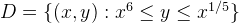# Double integrals 2

#### Petrus

##### Well-known member
Hello MHB,
I would like to have tips how to solve the x limits for this problem,

thereRegards,

#### Klaas van Aarsen

##### MHB Seeker
Staff member
Hello MHB,
I would like to have tips how to solve the x limits for this problem,
thereRegards,
Your area D is bounded by the 2 curves $y=x^6$ and $y=x^{1/5}$.
Where do they intersect?
Or put differently, can you solve that set of 2 equations?

#### Petrus

##### Well-known member
Your area D is bounded by the 2 curves $y=x^6$ and $y=x^{1/5}$.
Where do they intersect?
Or put differently, can you solve that set of 2 equations?
hmm $$\displaystyle x^6=x^{1/5} <=> x_1=1 x_2=0$$

#### Klaas van Aarsen

##### MHB Seeker
Staff member
hmm $$\displaystyle x^6=x^{1/5} <=> x_1=1 x_2=0$$
Yep.
Those are the x limits in your problem.

#### Petrus

##### Well-known member
Yep.
Those are the x limits in your problem.
Hello I like Serena,
I forgot to say thanks, I solved it!For anyone who is intrested how to solve it:
$$\displaystyle \int_0^1\int_{x^6}^{x^{\frac{1}{5}}} x^4y^6 \ dydx$$
We can always use Fubini's theorem and take out the constant and integrate the y so we got

$$\displaystyle \int_0^1 x^4 \left[\frac{y^7}{7}\right]_{x^6}^{x^{\frac{1}{5}}}$$
$$\displaystyle \int_0^1x^4\frac{x^{\frac{7}{5}}}{7}-x^4\frac{x^{42}}{7} dx$$
use the rule $$\displaystyle x^m*x^n=x^{n+m}$$ and we can simplify to ( we can take out a constant as well):

$$\displaystyle \frac{1}{7}\int_0^1x^{\frac{27}{5}}-x^{46} dx$$
$$\displaystyle \frac{1}{7} \left[ \frac{5x^{\frac{32}{5}}}{32} - \frac{x^{47}}{47} \right]_0^1 = \frac{29}{1504}$$

Regards,